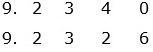# Introduction to Ordering Decimals

Decimals are arranged in either increasing or decreasing order. Ordering decimals is similar to ordering whole numbers.
For ordering decimals, certain rules are followed that are given below.

### Rules for ordering decimals

• Given a number of decimals, to order them, we first line them up vertically so that their decimal points are exactly one above other.

• If a number doesnot have a decimal point, we place the decimal at the end.

• More number of digits in a number does not necessarily mean it is a bigger decimal number (For example 0.42 and 0.416).

• Zeros are added to the right of the decimal numbers so that all of them have the same number of decimal places.

• The digits with same place value are compared starting from left in all the numbers.

• The number with a bigger digit in the same place value decides the bigger decimal number.

Compare the two decimals using the sign >, <, =

8.4, 8.416

### Solution

Step 1:

First we line up the numbers so that their decimal points are exactly one above another as followsStep 2:

Next we add zeros to the right of 8.4 to make both numbers have the same number of decimal places.Step 3:

Starting from leftmost column we compare the digits as follows.

The digits in ones place (8) and tenths place (4) in 8.400 and 8.416 are same. Next comparing the digits in hundredths place, we see that in 8.416, the digit is 1 where as in 8.400 it is 0. As 1 > 0. So, 8.416 > 8.400 or

8.4 < 8.416

Compare the two decimals using the signs >, <, =

9.234, 9.2326

### Solution

Step 1:

First we line up the numbers so that their decimal points are exactly one above another as followsStep 2:

Next we add a zero to the right of 9.234 to make both numbers have the same number of decimal places.Step 3:

Starting from leftmost column we compare the digits as follows.

The digits in ones place (9), tenths place (2) and hundredth place (3) in 9.2340 and 9.2326 are same. Next comparing the digits in thousandths place, we see that in 9.2340, the digit is 4 where as in 9.2326 it is 2. As 4 > 2,   9.2340 > 9.2326 or

9.234 > 9.2326